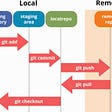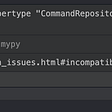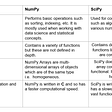# Solution in Python 3

Source Code Link:- ACM ICPC Team (github.com)

`def acmTeam(topic):    ans = []    for i in range(n):        for j in range(i+1, n):            know=0                        for a,b in zip(topic[i], topic[j]):                if a=='1' or b=='1':                   know+=1            ans.append(know)                     return [max(ans), ans.count(max(ans))]`

# Explanation

Firstly, we will declare an empty list called ans, Later on we will store the maximum number of topics known by a two-person team in it.

Then, we will use a nested for loop, the first for loop will iterate of the range of n using a variable i and the second for loop will iterate over range from i+1 to n using a variable j.

`for i in range(n):        for j in range(i+1, n):`

The nested loop, in essence will form the combinations of all possible teams. If value of n in a test case is 4, then the values of (i,j) will be (0,1), (0,2), (0,3), (1,2), (1,3), (2,3) which corresponds to all the possible combinations of two-person teams that can be formed with 4 people. Note that function parameter topic is a python list which is indexed from 0 thus, we iterate over the nested loop beginning from 0 itself.

Then, we declare a variable know and instantiate it to 0.

know = 0

Now, we will use another for loop and iterate over the zip of topic[i] and topic[j] using two variables a and b. The values of a and b are characters of string of binary digits which determine whether if a topic is known by a member of a two-person team.

If either the value of a equals to ‘1’ or b equals to ‘1’ we increment know by 1. This is because value of a and b determines the knowledge of a subject by the two members of the two-person team respectively. If either equals to ‘1’ then it means the two-person team knows the subject.

We represent the above logic in our code in the following way:-

`for a,b in zip(topic[i], topic[j]):    if a=='1' or b=='1':         know+=1`

After the end of the inner most for loop we append the know to ans.

`for i in range(n):        for j in range(i+1, n):            know=0                        for a,b in zip(topic[i], topic[j]):                if a=='1' or b=='1':                   know+=1            ans.append(know)`

At the end of the program, we return maximum value of ans which corresponds to maximum number of topics that can be known by a two person-team and count of maximum value of ans in ans in the form of a list.

return [max(ans), ans.count(max(ans))]

--

--

--

## More from Shounak Lohokare

pretending to teach is one of the best way to learn.

Love podcasts or audiobooks? Learn on the go with our new app.

## Automating LVM(Logical Volume Management) partition using python script.## Best Server Monitoring Software Tools Free and Paid in 2022## Python Training — Introduction — En Version## Ease of Building UI Elements in Unity!## Player -Color, Movement @ Unity Part 1## Semantic Docker with Semantic Release, Docker, and Travis CI## GIT & GITHUB IN 8 HOURS## Shounak Lohokare

pretending to teach is one of the best way to learn.

## Python subclassing and dependency inversion without violating Liskov substitution## SciPy-Tutorial## How To Make A Contact Book Application using Python (Part 2— Adding The CRUD Functionality)